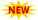## 23 September 2013

### MA2211 / MA31 / 10177 MA301 / 80100008 / MA1201A - Transforms and Partial Differential Equations - TPDE / M3 - Unit 5 - Notes

Anna university

Third Semester

MA2211 / MA31 / 10177 MA301 / 80100008 / MA1201A
Transforms and Partial Differential Equations

Regulation - 2008

Unit 5 - Notes

Semester: 3

Year: 2

Subject code:  MA2211 / MA31 / 10177 MA301 / 80100008 / MA1201A

Subject Title: Transforms and Partial Differential Equations

Type: Handwritten Notes

Number of Pages:19

Attachment format : Pdf

Version: Written Scanned Version

Regulation: 2008

Content: 3rd semester  - MA2211 / MA31 / 10177 MA301 / 80100008 / MA1201A - Transforms and Partial Differential Equations - Unit 5 Notes.

Submitted by: Namasi damcrazy

View 3rd semester  MA2211 / MA31 / 10177 MA301 / 80100008 / MA1201A Transforms and partial differential equations unit 5 Notes before download

Download a copy of 3rd semester  MA2211 / MA31 / 10177 MA301 / 80100008 / MA1201A Transforms and partial differential equations Unit 5 Notes

Search Terms:
TPDE Notes, Transforms and partial differential equations Notes, Anna University 3rd semster TPDE,
Anna University 3rd semester Transforms and partial differential equations , TPDE Formula Material,
Transforms and partial differential equations formula material, TPDE important Questions,Transforms and Partial Differential Equation question papers and question bank,Transforms and Partial Differential Equation previous year question papers,Tpde question papers,Transforms and Partial Differential Equation question papers,Transforms and Partial Differential Equation, Tpde notes, Maths 3 question papers, maths 3 notes,
M3 notes, M3 question papers, TPDE,  TPDE Formula,  M3 formula collection,  MA2211 Formula materials, MA2211 important formula,  TPDE important formula,  M3 important formula, Anna University Notes, M3 handwritten Notes, M3 Scanned Notes, TPDE Scanned NotesNeed more material ? Visit www.csetube.in